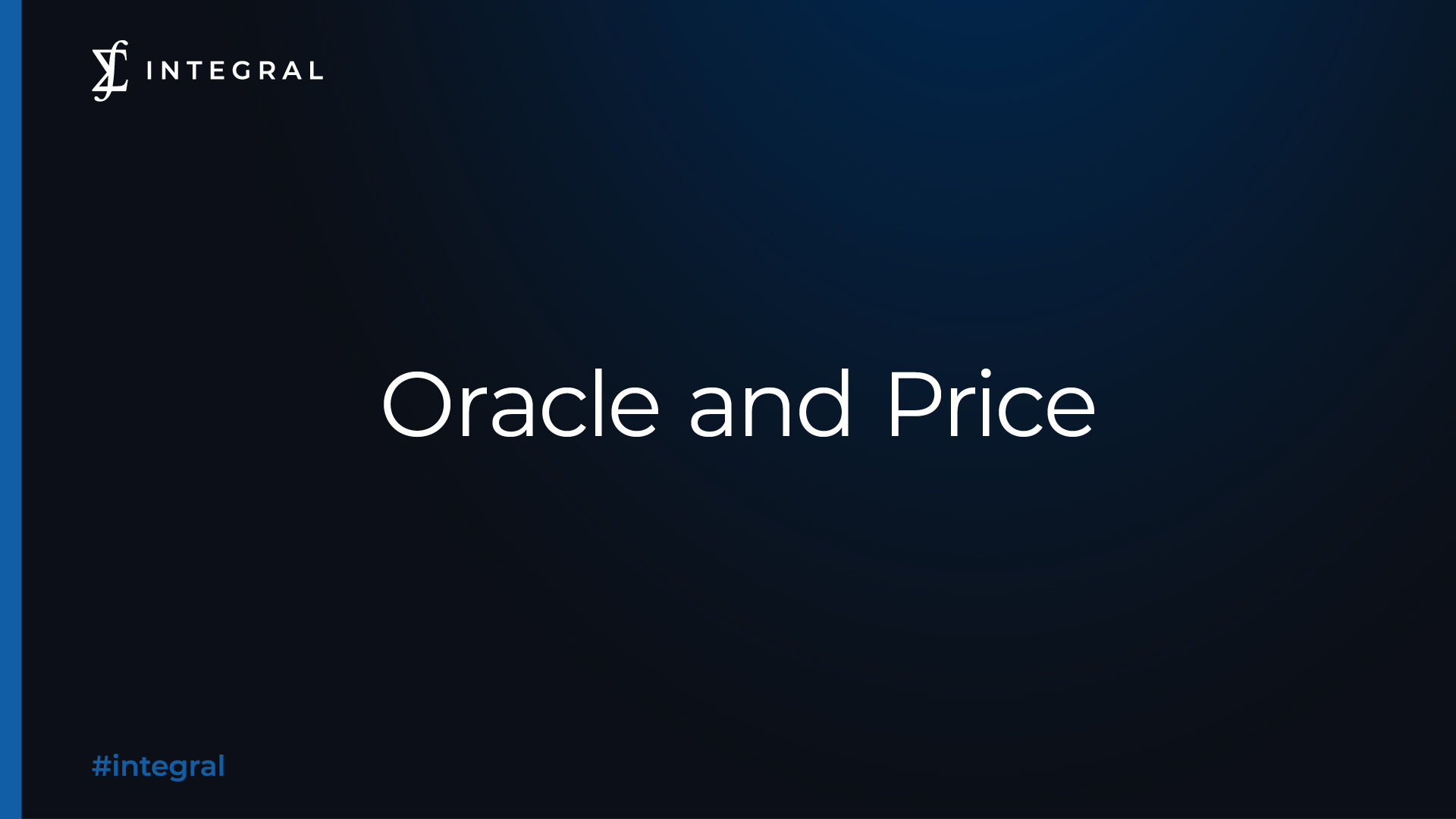Back to BlogThis blog postexplains the working mechanism of Integral TWAP's oracle, and summarizes how the protocol make sure that the time-weighted average price we use is fair, reliable, and hard to manipulate.

What's the role of oracle in Integral TWAP?

Oracle in the TWAP protocol is a smart contract that holds the reference to the external price oracle and contains the math that swaps involve (given tokenIn calculate tokenOut etc).

Currently TWAP only supports Uniswap v2 oracle.

What is unique about Integral TWAP and its oracle?

DEXs like Uniswap relies on curves to deduce price between two assets based on their amounts in the pool. A large swap will both increase and decrease the amount of assets, thus changing the ratio/price substantially and resulting price impact.

This does not apply to Integral TWAP, whose execution prices is solely determined by the oracle. If there is enough capital in the liquidity pool, your order will be completely filled with 1 SINGLE TWAP, without experiencing any price impact.

Which oracle does Integral TWAP use?

Integral TWAP uses Uniswap v2 as price oracle.

What makes Uniswap v2 qualified for being the oracle for Integral TWAP?

Arbitrageurs help Uniswap v2's price stay close to the market price

Since arbitrageurs will trade with Uniswap if the marginal price offered by Uniswap is incorrect (by a sufficient amount to make up for the fee), the price offered by Uniswap tends to track the relative market price of the assets.

A hard-to-manipulate design

Uniswap v2 improves its oracle functionality by measuring and recording the price before the first trade of each block (or equivalently, after the last trade of the previous block). This price is more difficult to manipulate than prices during a block.

Uniswap v2 accumulates this price (known as price accumulator), by keeping track of the cumulative sum of prices at the beginning of each block in which someone interacts with the contract. Each price is weighted by the amount of time that has passed since the last block in which it was updated, according to the block timestamp. This means that the accumulator value at any given time (after being updated) should be the sum of the spot price at each second in the history of the contract.

Example

Below is a simplified model of Uniswap v2's price accumulation in a ETH-USDC pool. Blue boxes represent the price accumulator in each block, while the white boxes represent prices of all swaps that take place in each block.

On t=0 sec, Block #1 is mined, and it contains multiple ETH-USDC swaps. The last swap of this block was executed with the price 1 ETH = 4001 USDC.

On t=12 sec, Block #2 is mined. The price accumulator of this block (denoted by a) will multiply the price of the last swap in Block #1 with the time interval between Block #1 and #2, which gives us 4001*12.

On t=24 sec, Block #3 is mined. After multiplying the price of the last swap in Block #2 with the time interval between Block #2 and #3, the price accumulator will also add its counterpart value in Block #2. Therefore, price accumulator in Block #3 is 4001*12+4004*12.

On t=37 sec, Block #4 is mined. Following the rules stated above, price accumulator of this block will be 4001*12+4004*12+4008*13.

To calculate the TWAP from t1 = 12 to t2 = 37, all we need to do is calculating (a(t2)-a(t1))/(t2-t1), which gives us 4006.08.

How Integral TWAP interacts with Uniswap v2 to calculate TWAP?

Once the order is submitted, it has to wait in the smart contract contract for 30 minutes before interacting with the pool. During this time, the protocol will query Uniswap v2 twice: once when the delay starts, and once when the delay ends.

After the delay time elapses, Integral TWAP will calculate the TWAP by doing the calculations above. If the actual amount of tokens you'll receive from TWAP is larger than or equal to the Minimum Received, your order will be executed at TWAP, and you will receive any positive slippage incurred. Otherwise it will be reverted to protect your funds from excessive slippage.

Minimum Received = Estimated Amount * (1 - Slippage Tolerance)

Why Integral TWAP Uses Uniswap v2 as Oracle instead of v3?

Uniswap v2 and v3 have different distribution of liquidity over price ranges:

• v2 has "constant liquidity" at every price from (0, infinity)
• v3's liquidity distribution could be anything, such as having lower and/or upper price bounds beyond which there is no liquidity. This results in "liquidity holes" in any price range.

For v2, pool reserves completely determine oracle robustness. But for v3, only knowing the 2 reserves is not enough, and it still requires oracle users to know the liquidity shape to determine robustness.